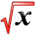# Average ValueThis article is part of the MathHelp Tutoring Wiki

Definition : Rolle's Theorem

f is a continuous function defined on the closed interval [a,b], is differentiable on the open interval (a,b), and f(a)=f(b)

Then, there exists a value "c" in the interval (a,b)such that f'(c)=0

If f is a continuous function defined on the interval [a,b]

The average of f(x) is : Average =${\frac {1}{b-a}}$baf(x)dx

Example 1 :

Find the average value of f(x) = $e^{x}$for 0 greater than equal to x and x less than equal to 2.
Solution :
Average = ${\frac {1}{2-0}}$20f(x)dx
=${\frac {1}{2}}$20$e^{x}$dx
=${\frac {1}{2}}$[$e^{x}$]20
=${\frac {1}{2}}$[$e^{2}-e^{0}$]
=${\frac {1}{2}}$[$e^{2}-1$]

Example 2 :

Find the average value of f(x) = $sqrt{x}$given the interval 0 greater than equal to x and x less than equal to 9.
Solution :
Average = ${\frac {1}{9-0}}$90$sqrt{x}$dx
=${\frac {1}{9}}$90$sqrt{x}$dx
=${\frac {1}{9}}$(${\frac {2}{3}}x^{\frac {3}{2}}$) |90
=${\frac {1}{9}}$(${\frac {2}{3}}9^{\frac {3}{2}}$- 0)
=${\frac {1}{9}}$(${\frac {2}{3}}$x 27)
=2

Example 3 :

Find the consumer's surplus for p = 50 - 0.06$x^{2}$, the demand curve, with x = 20.
Solution :
The price is:
B = 50 - 0.06$(20)^{2}$= 50 - 24 = 26
The consumer's surplus is:
200$[(50-0.06x^{2})-26]$dx
=∫200$(24-0.06x^{2})$dx
=$(24x-0.02x^{3})$|200
=24(20) - $0.02(20)^{3}$= 480 - 160
= 320